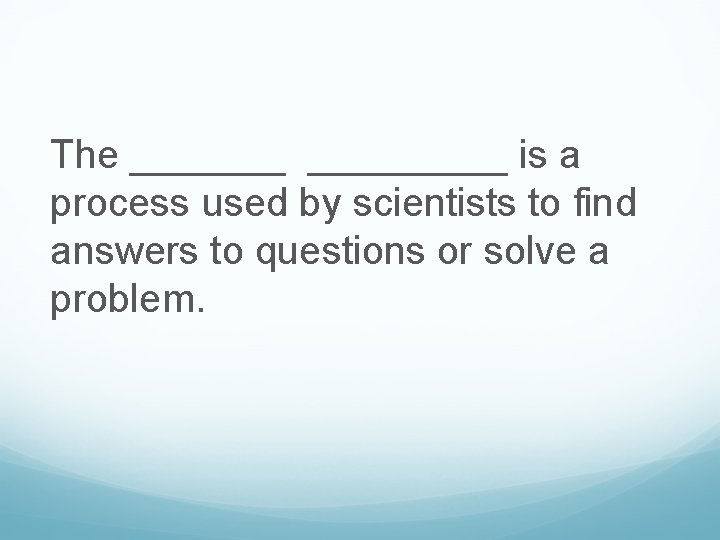# Scientific Method Test Review What is the six

• Slides: 94Scientific Method Test Review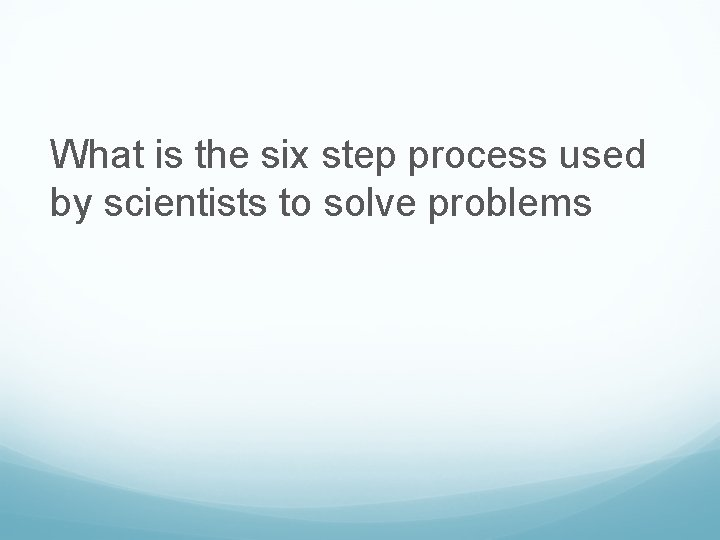What is the six step process used by scientists to solve problems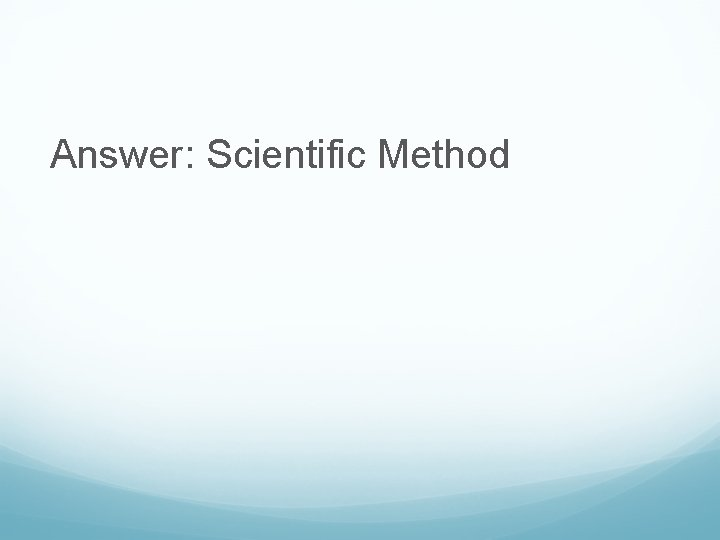What is an aspect of an experiment that changes as a result of the experiment and not because the scientist purposely changed it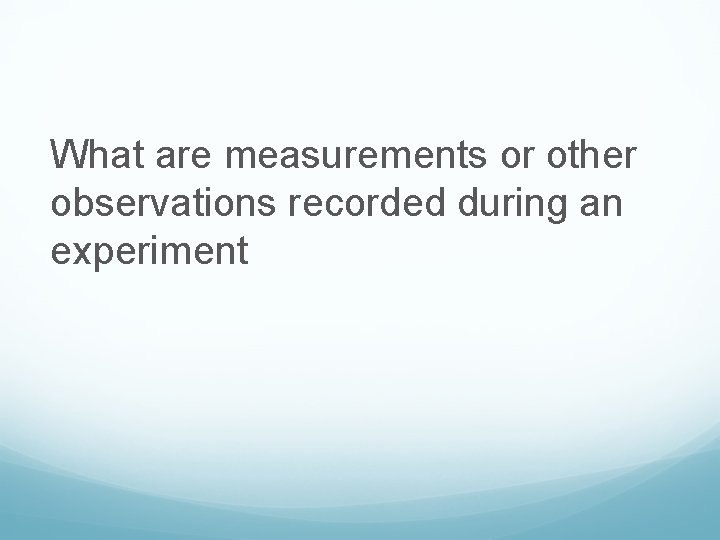What are measurements or other observations recorded during an experiment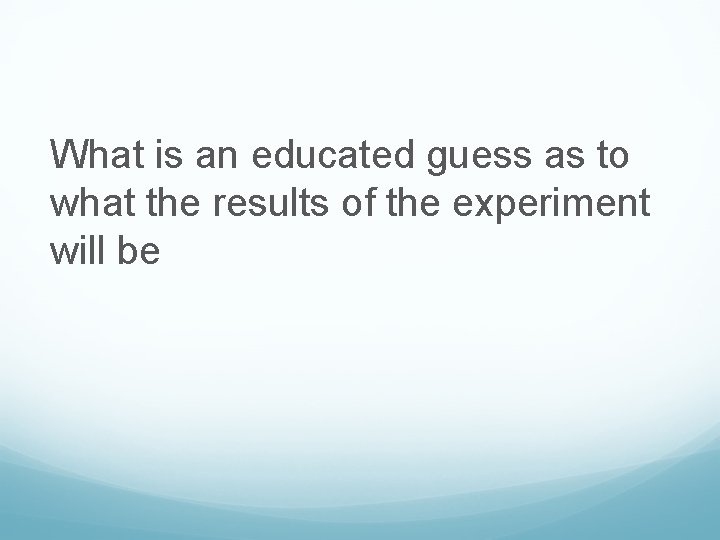What is an educated guess as to what the results of the experiment will beWhat do we call results of an experiment reached after analyzing the data collectedWhat is a fact that can be proven to be true using the five sensesWhat is the purpose of the experiment; the question the scientist is trying to find the answer to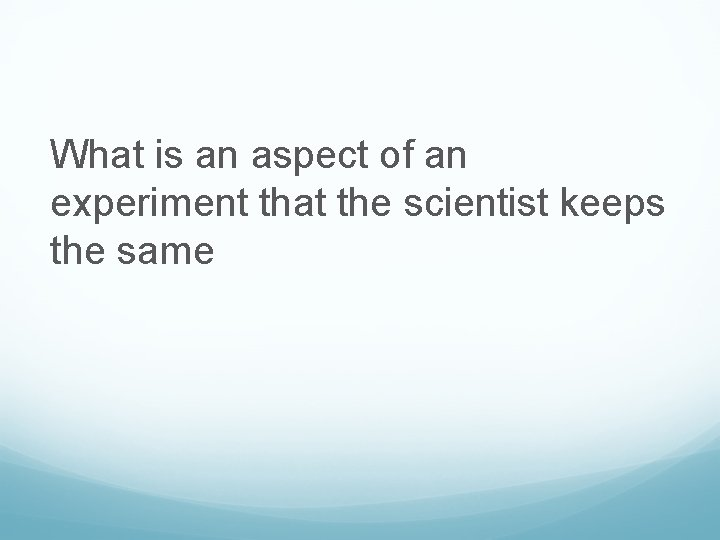What is an aspect of an experiment that the scientist keeps the sameWhat is the process used to investigate the problem using hands-on methods; follows a set of specific procedures or instructions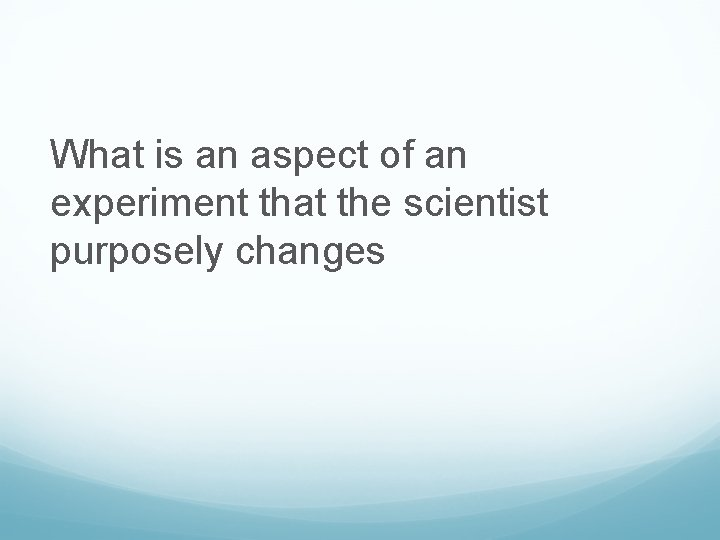What is an aspect of an experiment that the scientist purposely changesWhat graph would be used to represent percentages?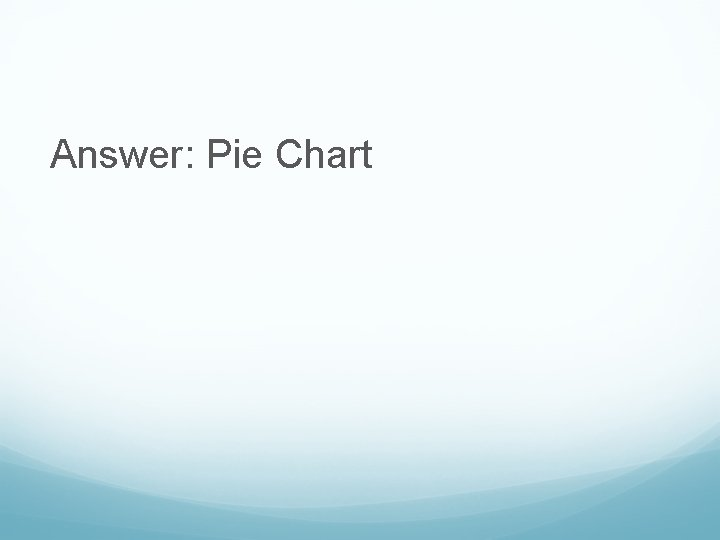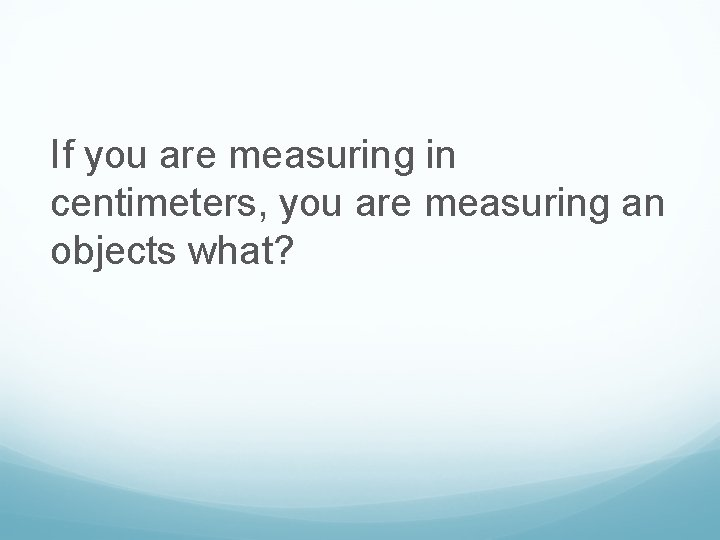If you are measuring in centimeters, you are measuring an objects what?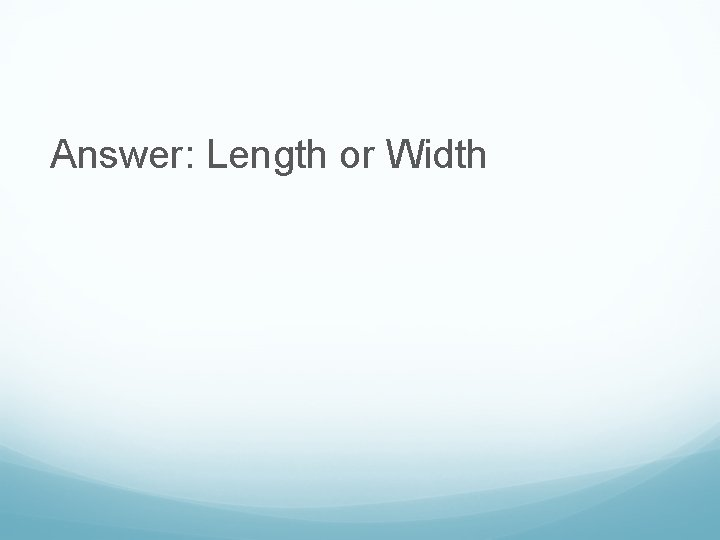Answer: Length or Width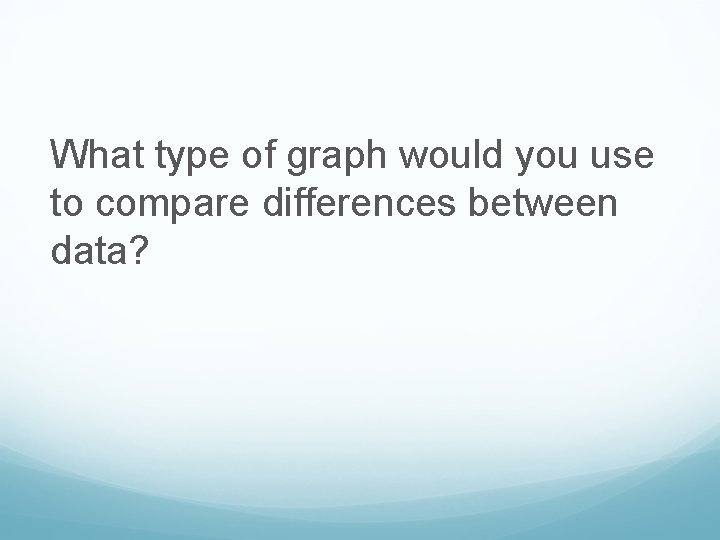What type of graph would you use to compare differences between data?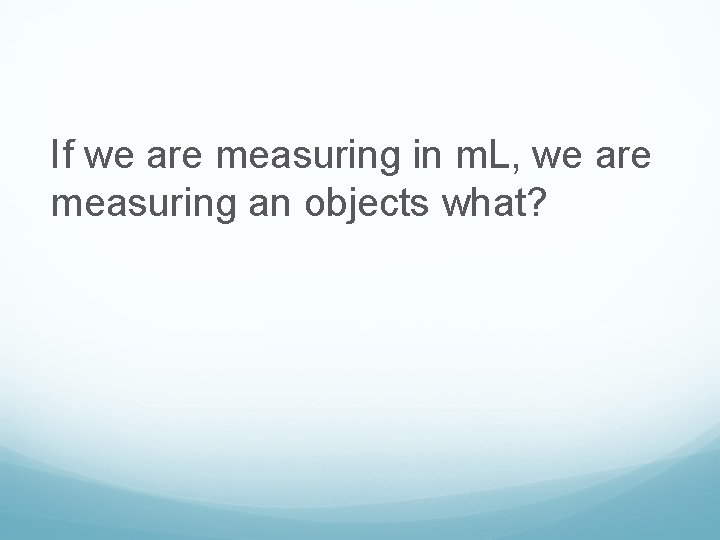If we are measuring in m. L, we are measuring an objects what?If we want to graph a change in data over a period of time, what graph would we use to display this?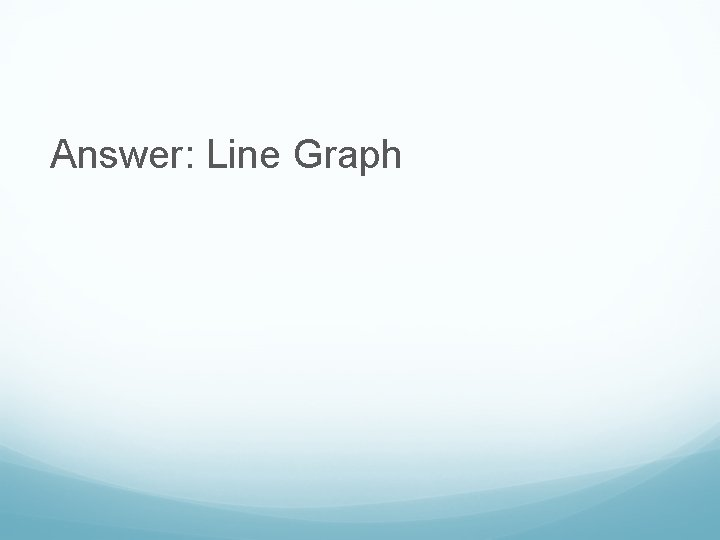If we are measuring in grams, we are measuring an objects what?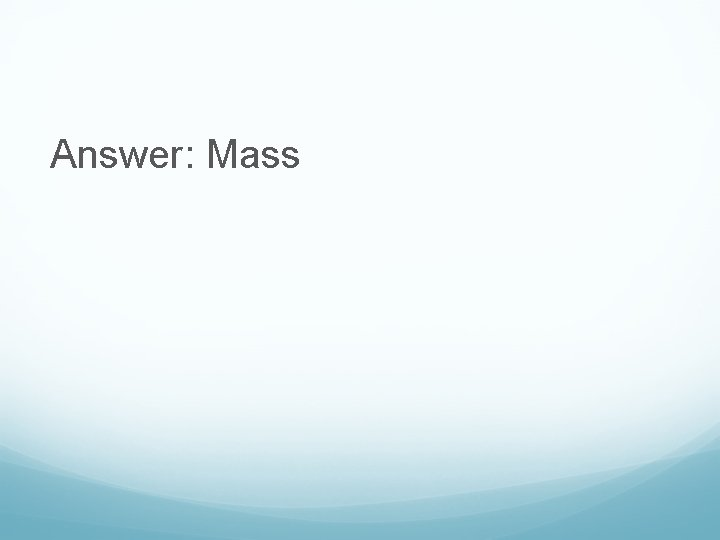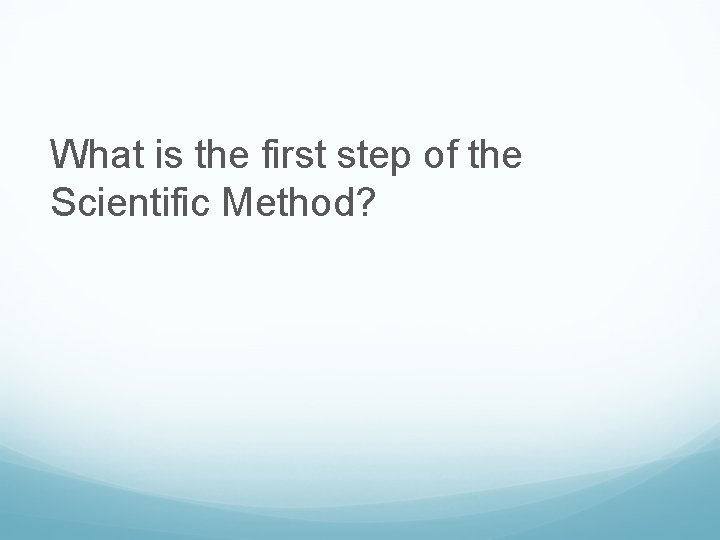What is the first step of the Scientific Method?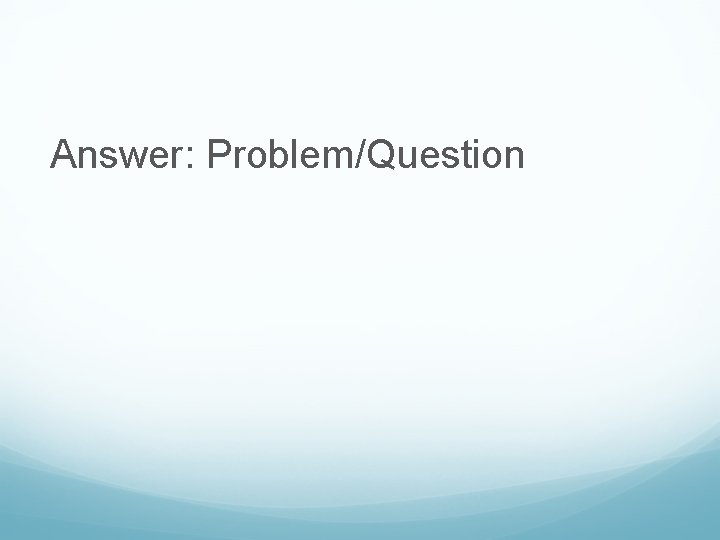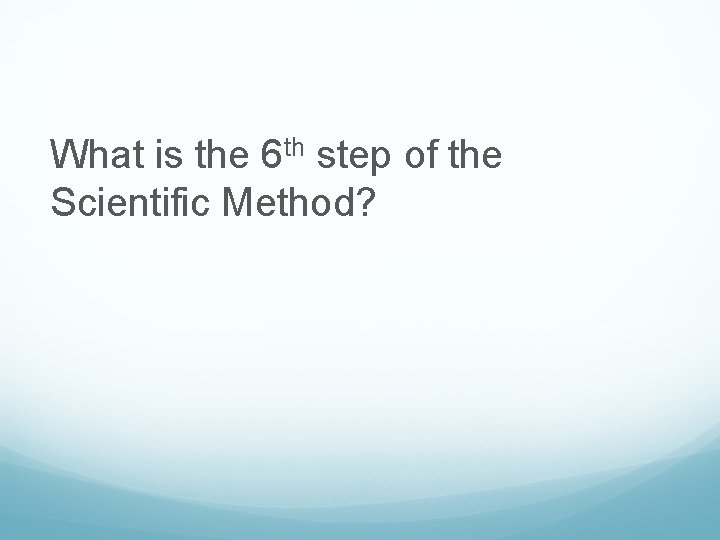What is the 6 th step of the Scientific Method?What is the 4 th step of the Scientific Method?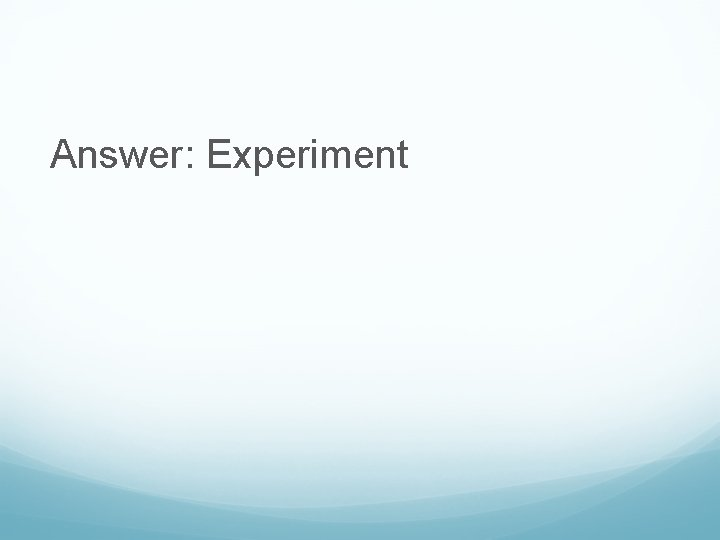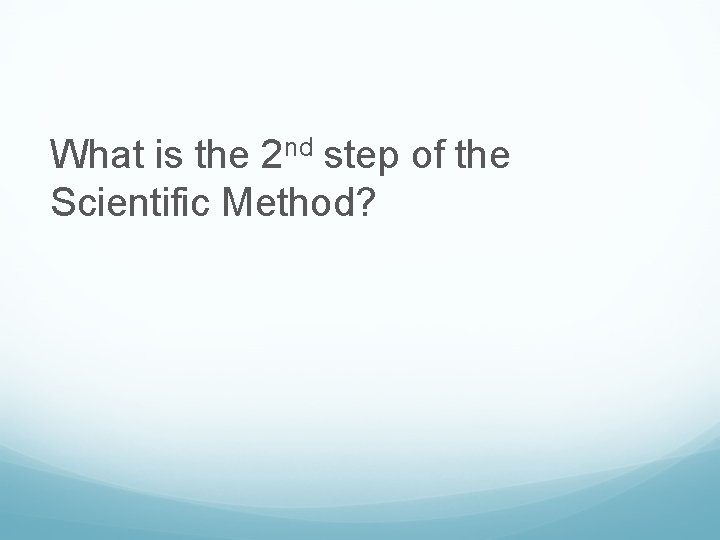What is the 2 nd step of the Scientific Method?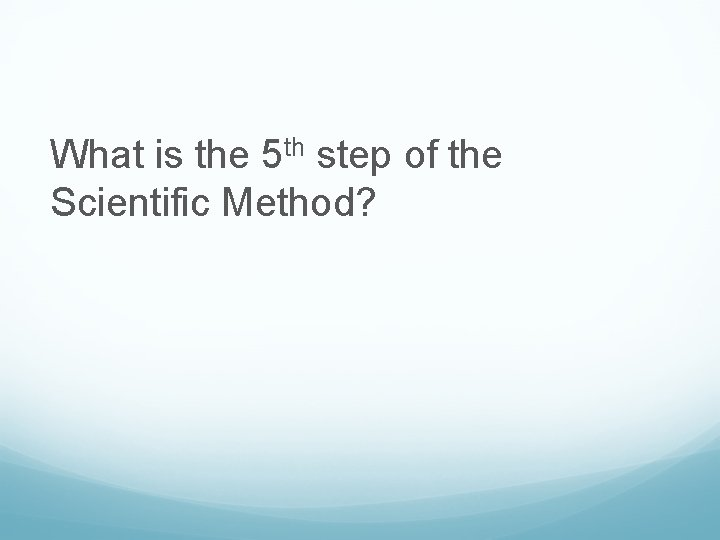What is the 5 th step of the Scientific Method?Answer: Analyze and Record Data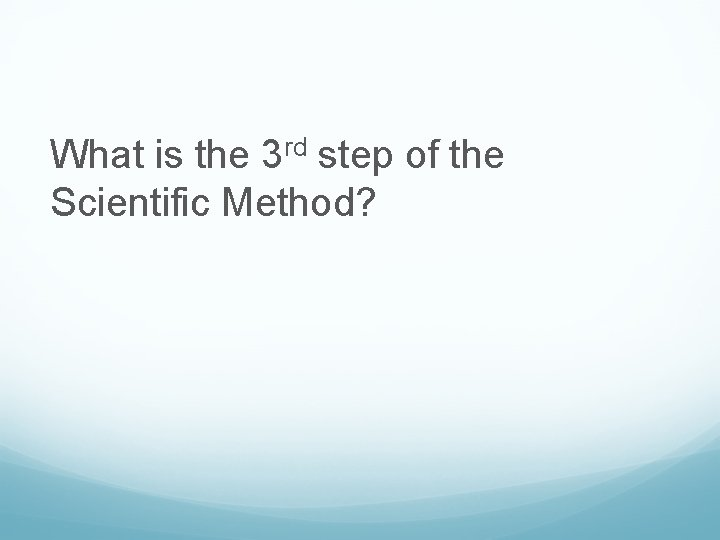What is the 3 rd step of the Scientific Method?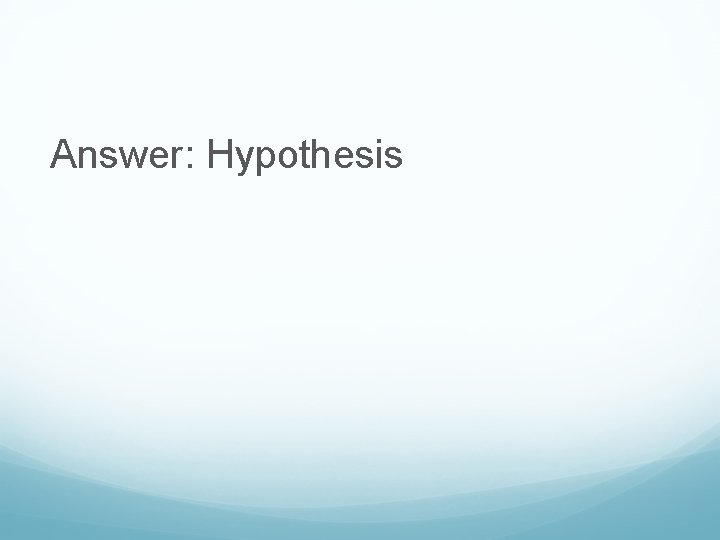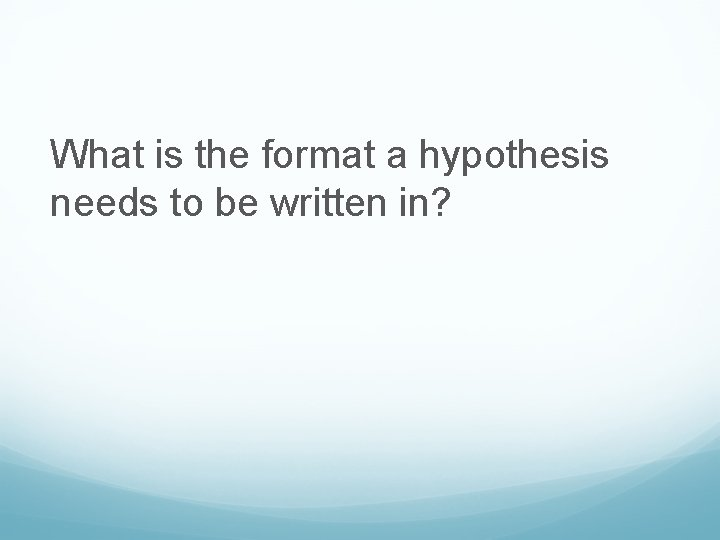What is the format a hypothesis needs to be written in?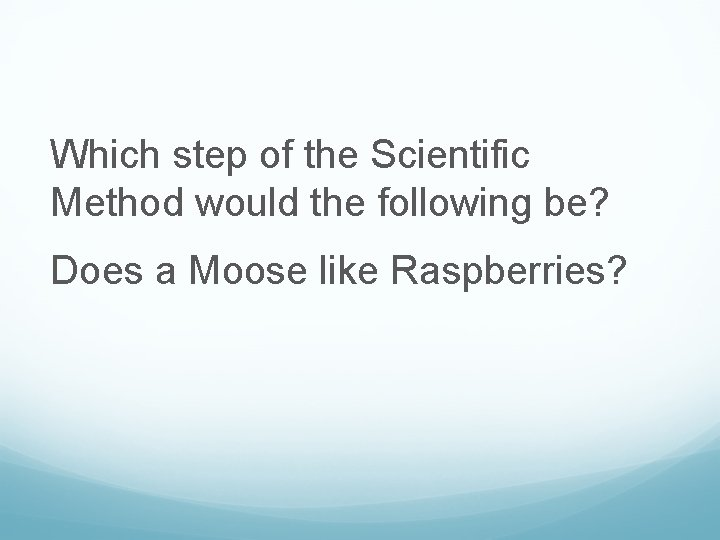Which step of the Scientific Method would the following be? Does a Moose like Raspberries?Answer: Step 1 Question/ProblemWhich step of the Scientific Method would the following represent? If raspberries are placed in a bowl in a moose’s feeding area, then he will not eat them.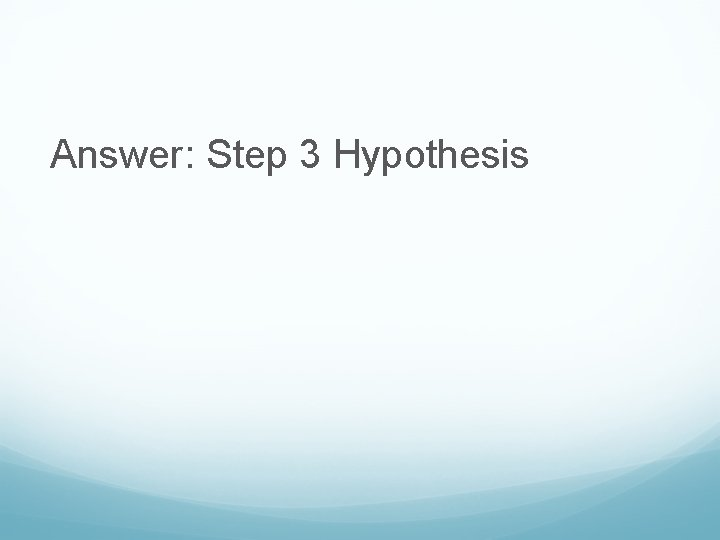Answer: Step 3 HypothesisWhich step of the Scientific Method would the following be a part of? Locate a moose. Place a bowl of raspberries in his feeding area. Observe for several feedings. Record the data.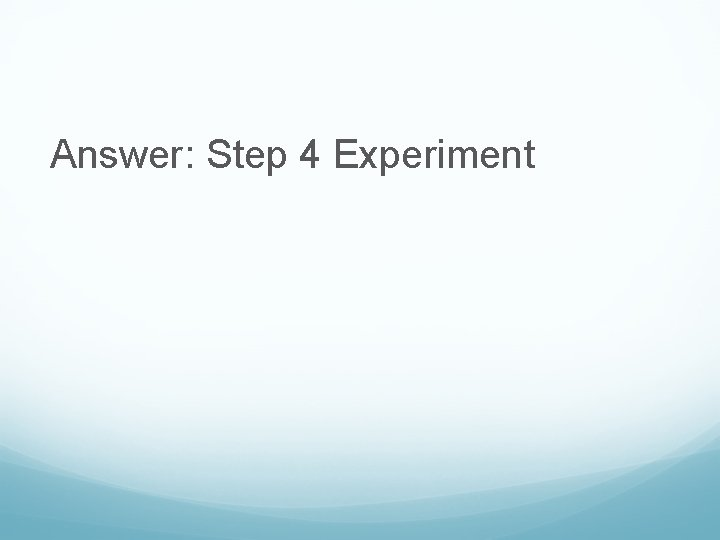Answer: Step 4 Experiment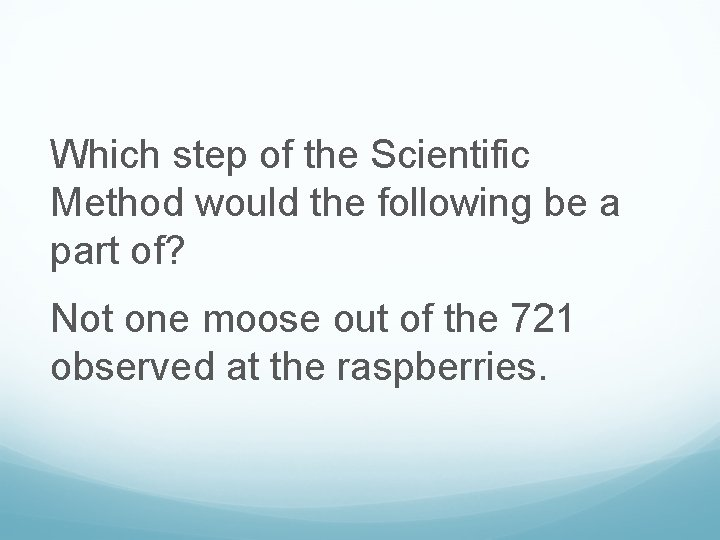Which step of the Scientific Method would the following be a part of? Not one moose out of the 721 observed at the raspberries.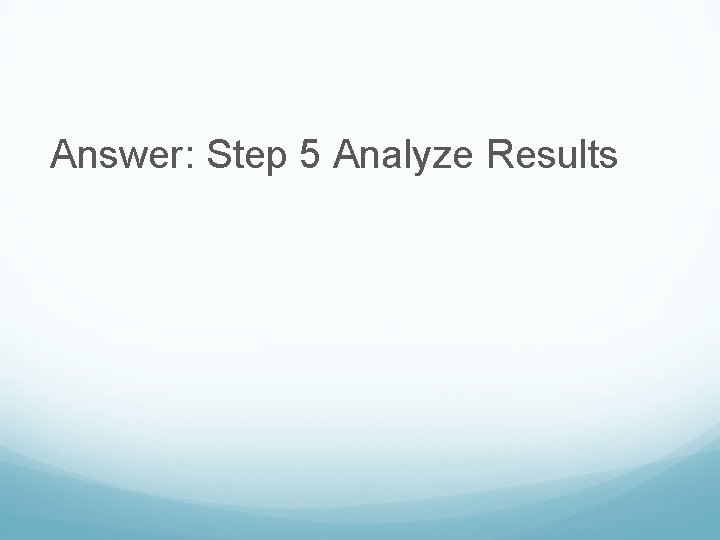Answer: Step 5 Analyze ResultsWhich step of the Scientific Method would the following represent? To sum up, a moose does not like raspberries.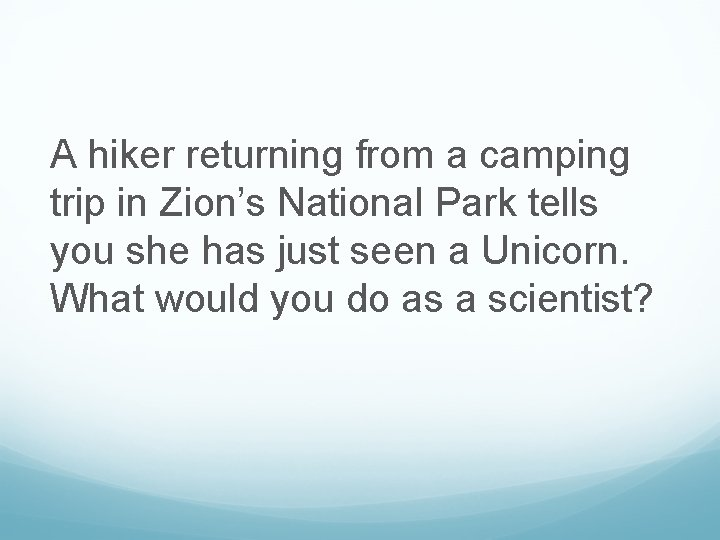A hiker returning from a camping trip in Zion’s National Park tells you she has just seen a Unicorn. What would you do as a scientist?Which step of the scientific method do scientist test their hypothesis?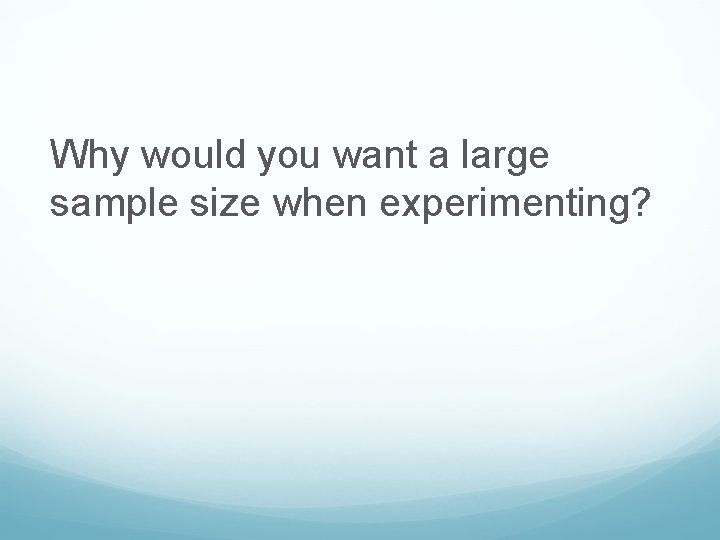Why would you want a large sample size when experimenting?Answer: to have more representative data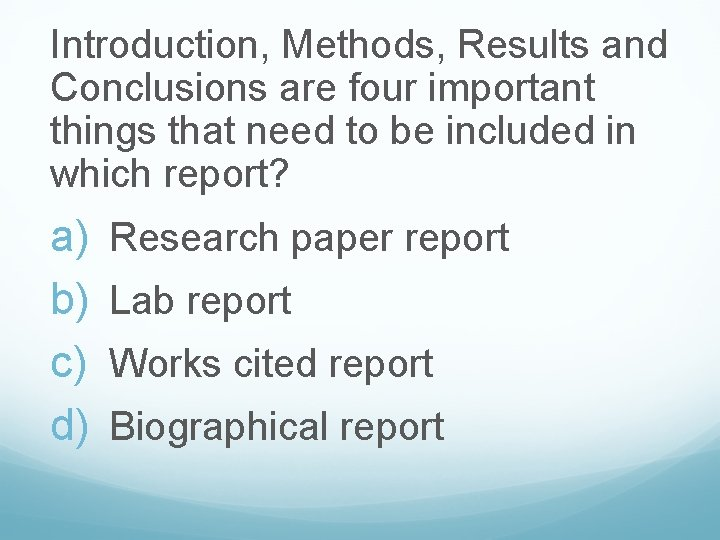Introduction, Methods, Results and Conclusions are four important things that need to be included in which report? a) b) c) d) Research paper report Lab report Works cited report Biographical report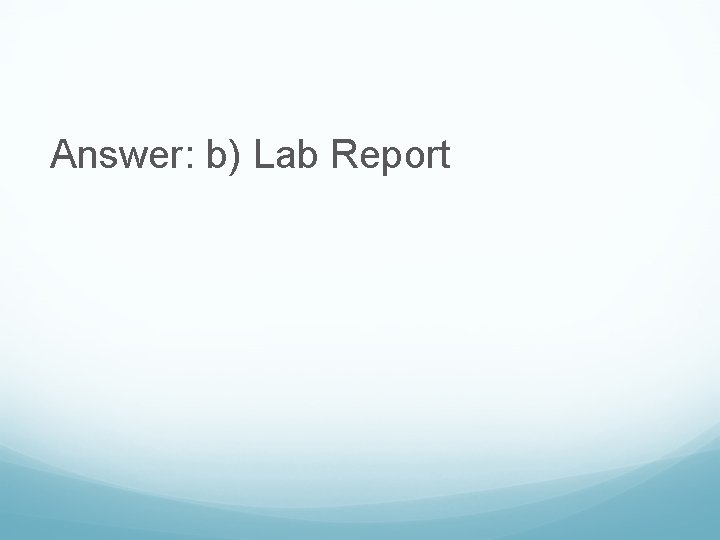Answer: b) Lab ReportCrossword Section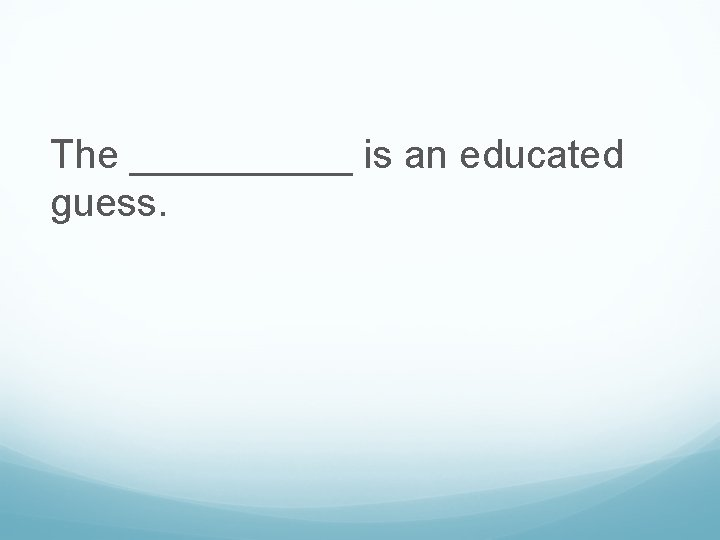The _____ is an educated guess.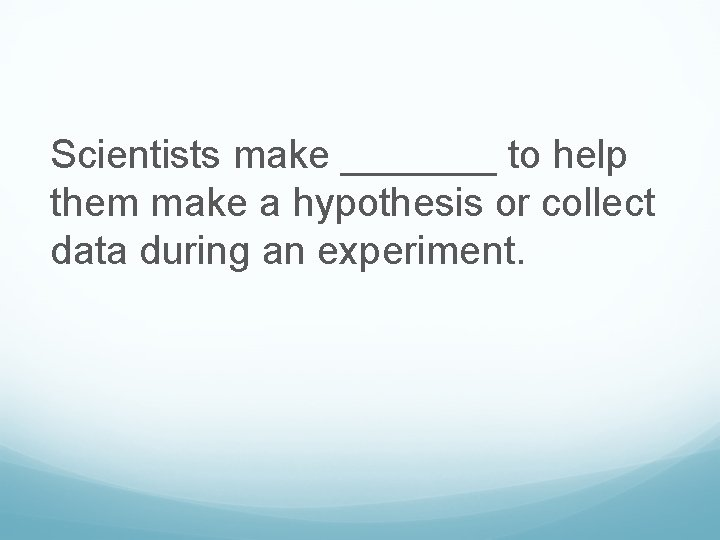Scientists make _______ to help them make a hypothesis or collect data during an experiment.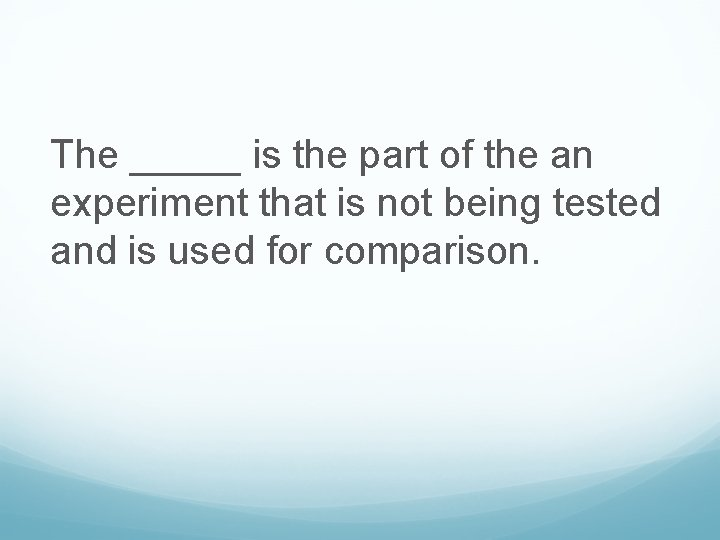The _____ is the part of the an experiment that is not being tested and is used for comparison.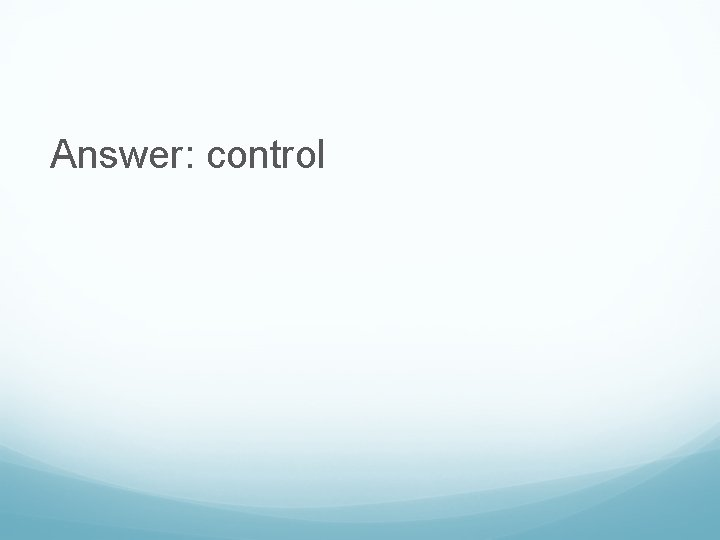Sometimes scientists make a mistake, or _______ and need to do an experiment again.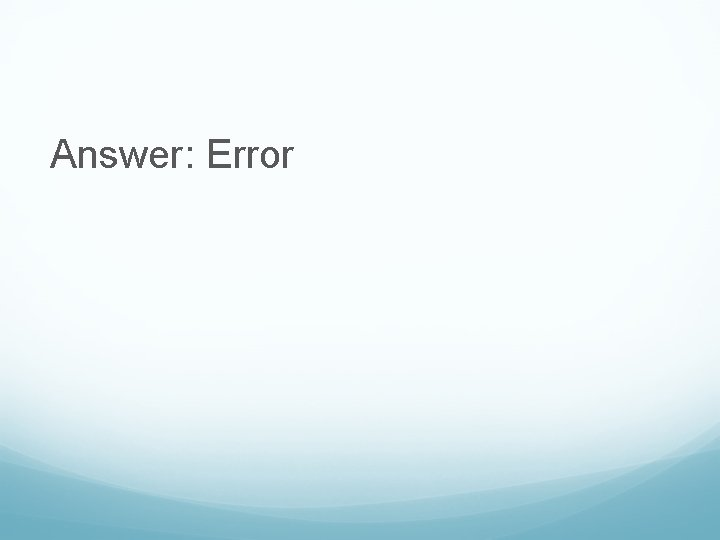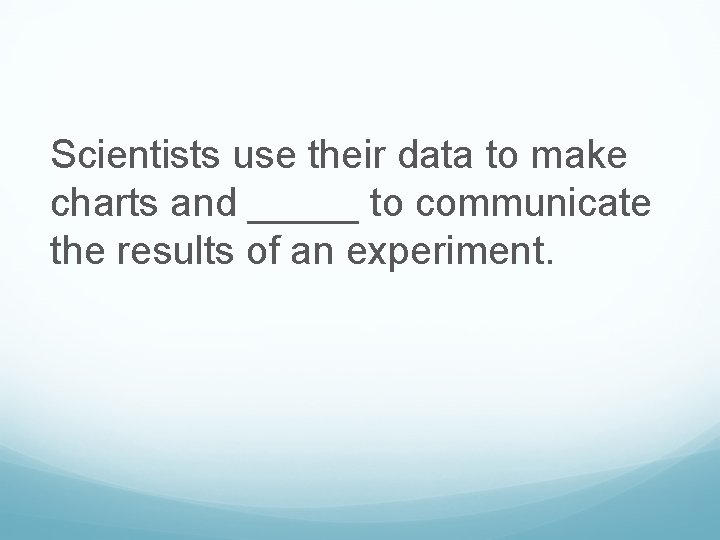Scientists use their data to make charts and _____ to communicate the results of an experiment.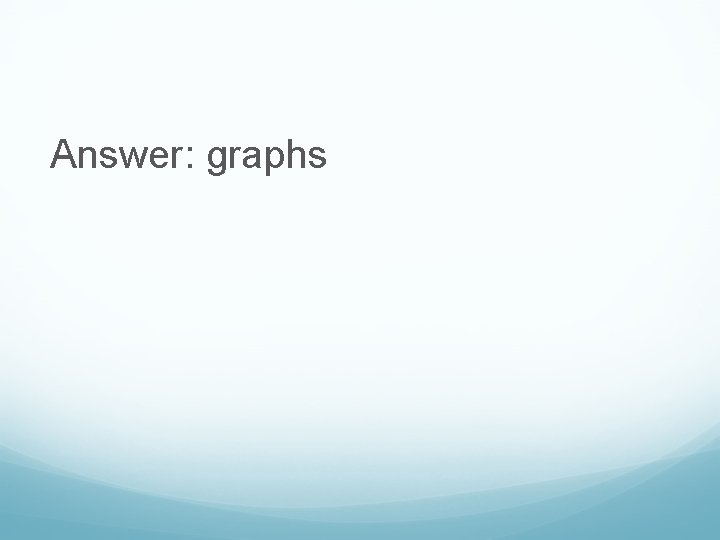The ____ describes the steps you use during an experiment.After an experiment, scientists write a _______ which summarizes their experiment and results.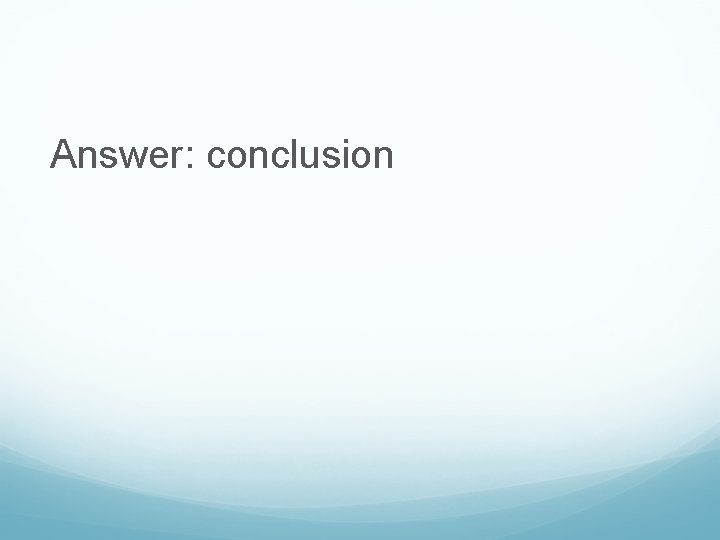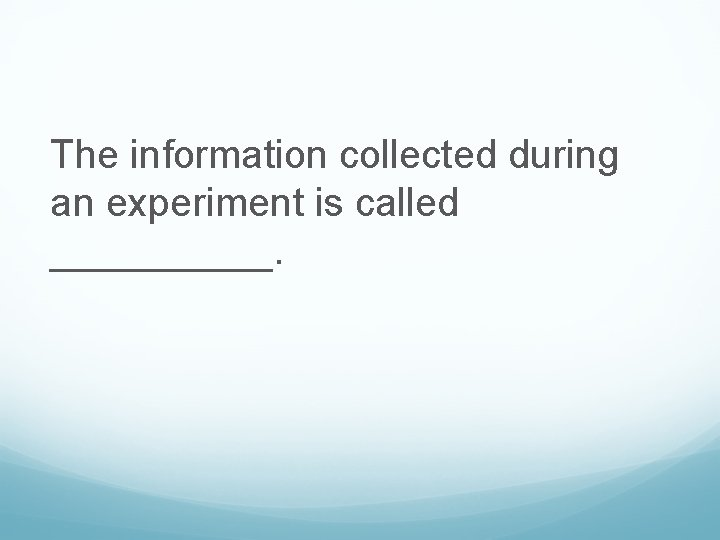The information collected during an experiment is called _____.After the scientist makes a hypothesis, the perform an _____ to collect data.The _____ variable is the part of the experiment that is being tested or the part that is changed by the person doing the experiment.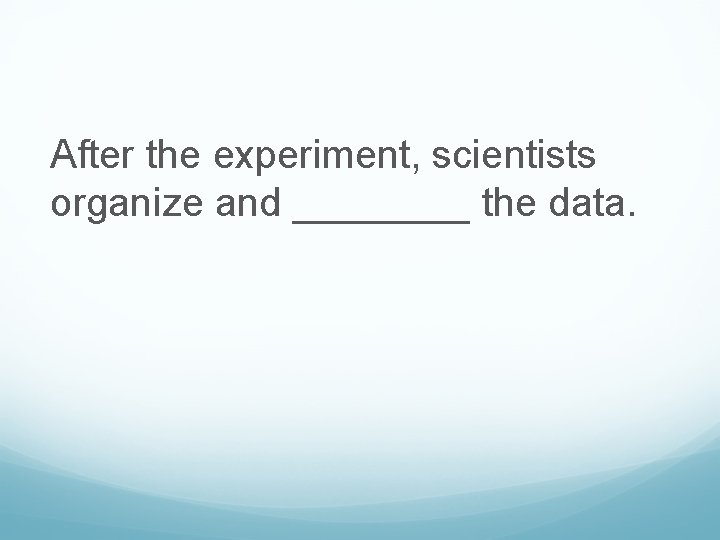After the experiment, scientists organize and ____ the data.The _____ variable is the part of the experiment that is affected by the independent variable.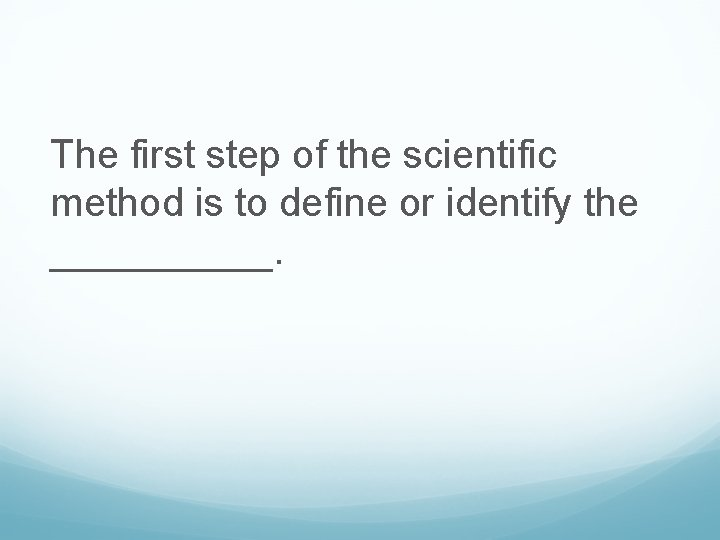The first step of the scientific method is to define or identify the _____.